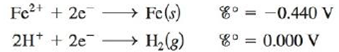# A galvanic cell is based on the following half-reactions: where the iron compartment contains an iron electrode and [Fe 2+ ] = 1.00 × 10 -3 M and the hydrogen compartment contains a platinum electrode, P H 2 = 1.00 atm , and a weak acid, HA, at an initial concentration of 1.00 M . If the observed cell potential is 0.333 V at 25°C, calculate the K a value for the weak acid HA.### Chemistry: An Atoms First Approach

2nd Edition
Steven S. Zumdahl + 1 other
Publisher: Cengage Learning
ISBN: 9781305079243

#### Solutions

Chapter
Section### Chemistry: An Atoms First Approach

2nd Edition
Steven S. Zumdahl + 1 other
Publisher: Cengage Learning
ISBN: 9781305079243
Chapter 17, Problem 145CP
Textbook Problem
15 views

## A galvanic cell is based on the following half-reactions:where the iron compartment contains an iron electrode and [Fe2+] = 1.00 × 10-3 M and the hydrogen compartment contains a platinum electrode, P H 2 =   1.00  atm , and a weak acid, HA, at an initial concentration of 1.00 M. If the observed cell potential is 0.333 V at 25°C, calculate the Ka value for the weak acid HA.

Interpretation Introduction

Interpretation:

The half-reaction taking place in a galvanic cell having Hydrogen as a cathode with a weak acid HA in the cathode compartment. The value of Ka for the weak acid HA is to be calculated.

Concept introduction:

Dissociation constant (Ka) is used for measuring the strength of an acid. It is basically dissociation constant with reference to an acid and base that are in equilibrium with respect to each other.

To determine: The value of Ka for the given weak acid.

### Explanation of Solution

Given,

The concentration of Fe2+ is 1.00×103M .

The pressure of Hydrogen gas is 1.00atm .

The concentration of weak acid HA is 1.00M .

The value of cell potential is 0.333V .

The reaction taking place at the cathode is,

2H++2eH2E°red=0.00V

The reaction taking place at the anode is,

Fe(s)Fe2++2eE°ox=0.440V

Add both the reduction and oxidation half-reactions as,

2H++2eH2(g)Fe(s)Fe2++2e

The final equation is,

2H++Fe(s)H2(g)+Fe2+

The value of E°cell is given as,

E°cell=E°ox+E°red

Where,

• E°ox is the oxidation potential of the electrode.
• E°red is the reduction potential of the electrode.

Substitute the values of E°ox and E°red in the above equation as,

E°cell=E°ox+E°red=0.440V+0.00V=0.440V_

The reaction involves the transfer of 2 moles of electrons.

The cell potential is calculated using the Nernst equation,

E=E°(0.0591n)log(Q)

Where,

• E is the cell potential.
• E° is the cell potential at the standard conditions.
• Q is the activity of the species in the cell.
• n is the number of electrons involved in the reaction.

Substitute the values of E° , Q, n , in the above equation as,

E=E°(0.0591n)log(Q)0.333=0.440(0.05912)log([Fe2+]pH2[H+]2)0.333=0.4400.02955log([1×103]1[H+]2)3.62=log([1×103]1[H+]2)

The equation is further simplified as,

3.62=log([1×103]1[H+]2)3

### Still sussing out bartleby?

Check out a sample textbook solution.

See a sample solution

#### The Solution to Your Study Problems

Bartleby provides explanations to thousands of textbook problems written by our experts, many with advanced degrees!

Get Started

Find more solutions based on key concepts
By law, food labels must name the ingredients in descending order of predominance by weight and in ordinary lan...

Nutrition: Concepts and Controversies - Standalone book (MindTap Course List)

How can solar flares affect Earth?

Horizons: Exploring the Universe (MindTap Course List)

Which organelles are in the endomembrane system?

Human Biology (MindTap Course List)

What role do plasmids play in recombinant DNA procedures?

General, Organic, and Biological Chemistry

A frictionless plane is 10.0 m long and inclined at 35.0. A sled starts at the bottom with an initial speed of ...

Physics for Scientists and Engineers, Technology Update (No access codes included)

Express the units of the force constant of a spring in SI fundamental units.

Physics for Scientists and Engineers with Modern Physics